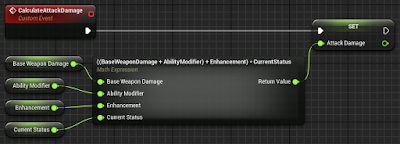## quarta-feira, 6 de abril de 2016

### Math expression

This is a special type of action that generates a sub-graph from a mathematical expression informed.

Input

• Expression: Expression that will be analyzed.
• Parameters defined in "Expression": For each variable name in the "Expression" is generated a new input parameter.

Output

• Return Value: Result of the expression.

Example Usage

The event below calculates the attack damage and stores the result in a variable. It used the "Math Expression" with the following expression:

(BaseWeaponDamage + AbilityModifier + Enhancement ) * CurrentStatus

Based on this expression the input parameters were generated.Click to enlarge